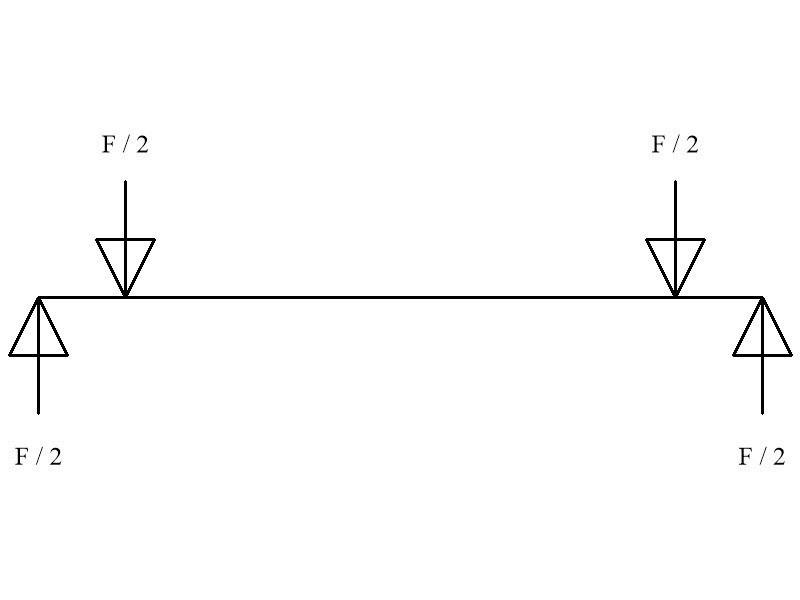# Bearing stress

For a hollow tube inside a hollow tube, with a pin passing through both and a force pushing down on the innermost tube, what would the bearing stress be on the holes (assuming tube wall thickness is equal)? Would the equivalent reaction force be F/4 since the pin is passing through 4 holes? So for example, say this is a jack stand with a 36,000 pound load on it. Would the bearing stress for each hole then be, 36,000/4 = 9000. Then bearing stress = F/td ? 9000/td Where t is wall thickness of tube and d is hole diameter?

Nidum